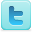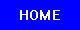Number Theory
Science Fair Projects
Ideas and Sample Projects by Grade Level

Middle / High School - Grades 7-12
P=Project   E=Experiment
Exploration and Analysis of Highly Divisible Numbers (antiprimes) [P]
Asymptotics of Character Sums [E]
Crank 0 Partitions and the Parity of the Partition Function [E]
Determine if a formula can be derived to find the number iterations it takes to reach zero or the Kaprekar Constant (6174) without going through the Kaprekar Routine. [E]
The Random Fibonacci Sequence [E]
Discover a method to multiply two numbers represented by the same quadratic form. [E]
Is Mathematics Discovered or Invented? [E]
Efficient True Random Number Generation [E]
Examine the correlation between the Fibonacci sequence and the spiral phyllotaxis, or leaf arrangement, of various plants occurring in nature. [E]
Test three random number generators (functions in Excel, C++, and Java) and evaluated the results using chi-square values from each experiment to determine which program was most likely to generate unbiased random numbers. [E]
Prove if Benford's Law applies to randomly generated numbers, and not just to numbers occuring from natural data sets. [E]
Experimental Verification of the Prime Number Theorem [E]
Build a binary marble adding machine [E]
What is a prime number? [P] [P]
The Efficiency of Prime-Testing Algorithms [E]
Pascal like Triangle, Sierpinski like Gaskets and Fibonacci like Sequences. [P]
A Mathematical Proof of a Relationship between Fibonacci and Lucas Numbers [E]
Pseudo-Random Numbers [E]
How to find perfect numbers. [E]
Is there a general divisibility rule, or pattern, that applies to any number? [E]
Observe the occurrences of Fibonacci numbers, sequences, the Fibonacci ratio, and the Fibonacci spiral in nature. [E]
Develope a circle map that represents the Collatz transformation and investigates its properties to make progress in proving the Collatz conjecture. [E]
Find out if there is a number that will disprove Goldbachs Conjecture [E]
What patterns are formed when number sequences are translated into different base systems and interpreted in base-10? [E]
Inverse Symmetry Pattern in the Multiplication Table [E]
Pi Science Fair Projects
R=Resource
Science Fair Projects Resources [R]
Citation Guides, Style Manuals, Reference [R]

 Mathematics Projects Math Topics Related Subjects Statistics & Probability Geometry & Trigonometry Applied Mathematics Games & Game Theory Number Theory Mathematics Learning Algebra & Calculus Computer Science Intelligence

 Science Fair Project Guide Home Science Fair Project Types The Scientific Method - How to Experiment The Display Board Topics, Ideas, Sample Projects